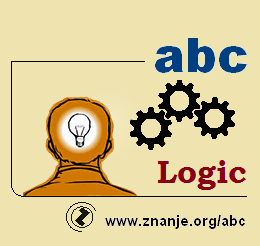abc Logic -
QUIZ - TEST

The program ensures that every chapter has an equal number of questions (base is 116 questions). With each new start of the quiz, 15 new questions are chosen from the base.

-QUIZ with images - (15 questions) - These questions have an image/animation that helps exlpain the question. After each question, you will be told whether your answer is correct or not.The DESCRIPTION link is located under the image/animation. There, an explanation can be found. Questions always come from the chapter for which the quiz is named.

-QUIZ without images - (15 questions) - After each question, you will be told whether your answer is correct or not.The DESCRIPTION link is located under the front image. There, an explanation can be found. Questions always come from the chapter for which the quiz is named.

- TEST with images - (15 questions) - These questions have an image/animation that helps exlpain the question. At the end of the quiz you are graded and links are given to explain your wrong answers.

- TEST without images - (15 questions) - At the end of the quiz you are graded and links are given to explain your wrong answers.

- TEST - (20 questions) - At the end of the test, you see your results. Questions always come from the chapter for which the test is named. Once you move past a question, you cannot go back. You are forbidden from changing your score or time. The color of the timer indicates the time past.

(Translation: Kelton Miller III8,Milos Simatovic III3,Anja Mitrasinovic III3 2017)QUIZ - Preparing - TEST abc Logika lessons abc Logika - Introduction - Quiz with images 1. Chapter abc Logika - Introduction - Quiz without images 1. Chapter abc Logika - Introduction - Preparing test with images 1. Chapter abc Logika - Introduction - Preparing test without images 1. Chapter abc Logika 2. Chapter lekcije abc Logika - Term - Quiz with images 2. Chapter abc Logika - Term - Quiz without images 2. Chapter abc Logika - Term - Preparing test with images 2. Chapter abc Logika - Term - Preparing test without images 2. Chapter abc Logika - 3. Chapter lekcije abc Logika - Sud - Quiz with images 3. Chapter abc Logika - Sud - Quiz without images 3. Chapter abc Logika - Sud - Preparing test with images 3. Chapter abc Logika - Sud - Preparing test without images 3. Chapter

Index